## LetsPlayMaths.Com

WELCOME TO THE WORLD OF MATHEMATICS

# Class-8 Factorisation

Introduction to Factorisation

Factors of Algebraic Expression

Concept of HCF

Methods of Factorisation of Polynomials

Factorisation by Taking out Common Factors

Factorising by Grouping of Terms

Factorising Using Algebraic Identities

Factorising difference of two squares by using algebraic identities

Factorisation Test

Factorisation Worksheets

## Introduction to Factorisation

We already learnt that composite number formed by the product of prime numbers. For example, 30 = 2 × 3 × 5, here, 2, 3, 5 are prime factors of 30. Similarly, the term of an algebraic expressions is formed as the product of factors.

Example 1. In (3xy + 4y)expression, the term 3xy is formed by the product of 3, x, y. So, 3, x, and y are factors of 3xy.

Example 2. In 2a2 (3a + 4b) = 6a3 + 8a2b. Here, 2a2 and (3a + 4b) are factors of (6a3 + 8a2b).

## Factors of Algebraic Expression

When an algebraic expression can be written as the product of two or more expressions, then each of these expressions is called factor of an algebraic expression.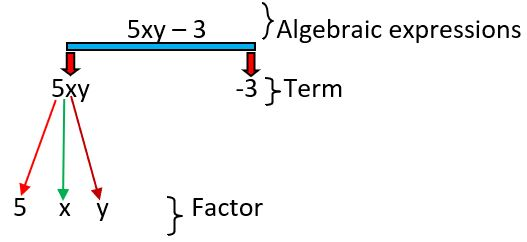The process of finding two or more expressions whose resultant product is an expression is called factorisation.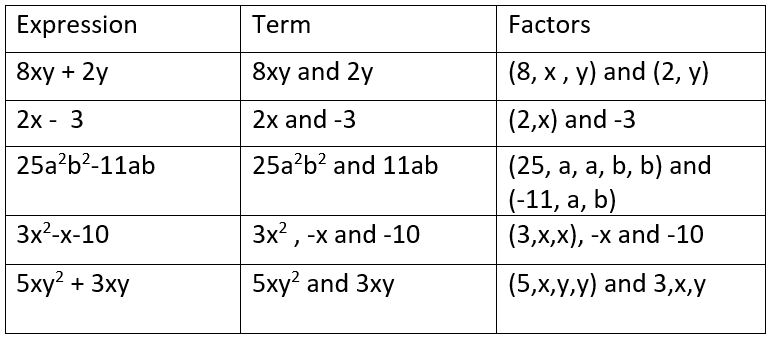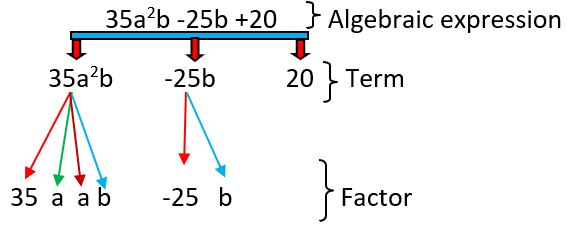Product of 3xy and (2xy − 5) results in 6x2y2 − 15xy, here the factors of (6x2y2 − 15xy) is 3xy and 2xy − 5.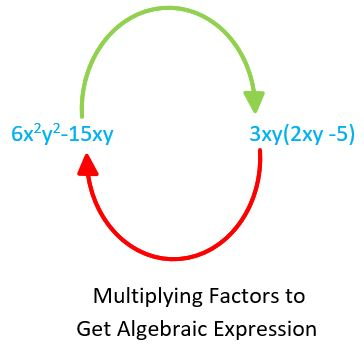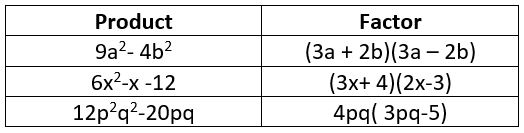## Concept of HCF

HCF of two or more polynomials means the largest common factor of the given polynomials, it is calculated by using following formula.

HCF of two or more monomial = (HCF of their numerical coefficients) × (HCF of their variable coefficient)

To find out the HCF of literal coefficient, we should do the product of each common variable raised to lowest power.

Example HCF of 8y2z2 and 4yz2.

Step-1 We calculate the HCF of numerical coefficient = HCF of 8 and 4 = 4

Step-2 We calculate the HCF of literal coefficient = HCF of y2z2 and yz3 = y × z2 = yz2

Hence, HCF of 8y2z2 and 4yz3 = 4 × yz2 = 4yz2

## Methods of Factorisation of Polynomials

1. Taking out common factors
2. Grouping
3. Using algebraic identities

## Factorisation by Taking out Common Factors

When we factorised the polynomial, we should follow the below process.

Step-1 Find out the HCF of all the terms of the given polynomial.

Step-2 Divide each term of the given polynomial by it's HCF.

Step-3 Enclose the quotient within the brackets.

Step-4 Keep the common factor outside the bracket.

Let's see some examples to understand the factorisation process.

Example 1. Factorise 363 − 12x2

Solution. Given expression is 36x3 − 12x2

First, we find HCF of 36x3 and 12x2

So, HCF of 36x3 and 12x2 = 12x2

Then divide (36x3 − 12x2) with 12x2 we get

(36x3 − 12x2)12x2 = (36x312x2) − 12x212x2

= (3x − 1)

Then keep 12x2 outside the (3x − 1)

= 12x2(3x − 1)

Hence, 36x3 − 12x2 = 12x2(3x − 1)

Example 2. Factorise 2x (z + 3y) + 7a(z + 3y).

Solution. First, find HCF of the 2x(z + 3y) and 7a(z + 3y) and the result is (z + 3y).

Divide each expression by z + 3y and keep it outside of the bracket.

Hence, 2x(z + 3y) + 7a(z + 3y) = 2x(z+3y)(z+3y) + 7a(z+3y)(z+3y)

= 2x + 7a

Then keep z+3y outside the bracket = (z + 3y)(2x + 7a)

Hence, 2x(z + 3y) + 7a(z + 3y) = (z + 3y)(2x + 7a)

## Factorising by Grouping of Terms

This method is possible only when the given polynomial contains an even number.

a) Arrange the terms of given expression in groups. Each group contain common factor.

b) Factorise each group.

c) Take out factor which is common to each other.

Example 1. Factorise the xy − xz + my − mz

Solution. xy − xz + my − mz = (xy − xz) + (my − mz)

= x(y − z) + m(y − z)

= (y − z)(x + m)

Example 2. Factorise the p4 + 2p3 + p + 2

Solution. p4 + 2p3 + p + 2 = (p4 + 2p3) + (p + 2)

= p3(p + 2) + 1(p + 2)

= (P + 2)(p3 + 1)

Example 3. Factorise pq + xy + px + qy.

Solution. In this type of expression, we do not group the term in pairs those have no common factor. So, we should first interchange the position of terms. Here we interchange the position of (xy) and (qy). Then we do the factorisation by grouping.

pq + xy + px + qy

= pq + qy + xy + px

= q(p+y) + x (p + y) = (p+q)(q + x)

Example 4. Factorise pq (m2 + n2) + mn(p2 + q2)

Solution. In this expression we should first multiply (pq) with(m2 + n2) and (mn) with(p2 + q2) by using distributive law over addition. Then do the factorisation by grouping method.

pq (m2 + n2) + mn(p2 + q2)

= pqm2 + pqn2 + mnp2 + mnq2 (interchange the position of mnp2 and pqn2)

= pqm2 + p2mn + pqn2 + mnq2

= pm(qm + pn) + qn(pn + qm)

= pm(pn + qm) + qn(pn + qm)

= (pn + qm)(pm + qn)

## Factorising Using Algebraic Identities

Polynomial can be factorised by using algebraic identities like (a + b)2 = a2 + 2ab + b2 and (a − b)2 = a2 − 2ab + b2 if polynomial is a perfect square.
In this case first we try to convert given polynomial into (a2 + 2ab + b2 ) or (a2 − 2ab + b2) form and then replace it by (a + b)2 and (a − b)2 respectively. Let's see some examples.

Example 1. Factorise X2 + 16x + 64

Solution. X2 + 16x + 64 = x2 + 2 × (x) × 8 + 82

= (x + 8)2, (here we use algebraic identities (a + b)2 formula.)

## Factorising difference of two squares by using algebraic identities

The polynomial in the form of difference of two squares, then it can be factorised by using the identity like a2 − b2 = (a + b)(a − b)

Example 1. Factorise 36x2 − 81y2

Solution. 36x2 − 81y2 = (6x)2 − (9y)2

= (6x + 9y)(6x − 9y).

## Class-8 Factorisation Test

Factorisation - 1

Factorisation - 2

## Class-8 Factorisation Worksheet

Factorisation Worksheet - 1

Factorisation Worksheet - 2

Factorisation Worksheet - 3